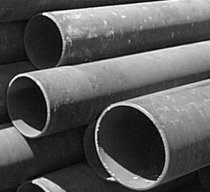Engineering ToolBox - Resources, Tools and Basic Information for Engineering and Design of Technical Applications!

# Pipe Formulas

## Pipe and Tube Equations - moment of inertia, section modulus, traverse metal area, external pipe surface and traverse internal area - imperial units

### Online Pipe Formula Calculator

outside diameter (in)

inside diameter (in) - (default values: STD 4 inches - Carbon, Alloy and Stainless Steel Pipes - ASME/ANSI B36.10/19

The calculator is based on the piping formulas and equations below.### Moment of Inertia

Moment of inertia can be expressed as

I  = π (do4 - di4) / 64
≈ 0.0491 (do4 - di4)                            (1)

where

I = moment of inertia (in4)

do = outside diameter (in)

di = inside diameter (in)

### Section Modulus

Section modulus can be expressed as

S  = 0.0982 (do4 - di4) / do                            (2)

where

S = section modulus (in3)

Section modulus is a geometric property for a given cross-section used in the design of beams or flexural members.

### Transverse Metal Area

Transverse metal area can be expressed as

Am  = π (do2 - di2) / 4                              (3)

where

Am = transverse metal area (in2)

### External Pipe Surface

External pipe or tube surface per ft of length can be expressed as

Ao  = π do / 12                              (4)

where

Ao = external  pipe surface area (ft2 per ft pipe)

### Internal Pipe Surface

Internal pipe or tube surface per ft of length can be expressed as

Ai  = π di / 12                            (5)

where

Ai = internal  pipe surface area (ft2 per ft pipe)

### Transverse Internal Area

Transverse internal area can be expressed as

Aa  = 0.7854 di2                       (6)

where

Aa = transverse internal area (in2)

### Circumference External

External circumference can be expressed as

Ce  = π do                      (7)

where

Ce =  external circumference (in)

### Circumference Internal

Internal circumference can be expressed as

Ci  = π di                       (8)

where

Ci =  internal circumference (in)

### Estimating Pipe Circumference and Section Area

Nominal Pipe Size
(in)
Circumference
(in)
Section Area
(sq.in.)
1/4 0.785 0.049
3/8 1.178 0.110
1/2 1.571 0.196
3/4 2.356 0.442
1 3.142 0.785
1 1/4 3.927 1.227
1 1/2 4.712 1.767
2 6.283 3.142
2 1/2 7.854 4.909
3 9.425 7.069
3 1/2 11.00 9.621
4 12.57 12.57
5 15.71 19.64
6 18.85 28.27
8 25.13 50.27
10 31.42 78.54
12 37.70 113.1
15 47.12 176.7

## Related Topics

• ### Dimensions

Sizes and dimensions of pipes and tubes, and their fittings - inside and outside diameter, weight and more.

## Related Documents

• ### ANSI Schedule 40 Steel Pipes - Dimensions

Internal and external diameters, areas, weights, volumes and number of threads for ANSI schedule 40 steel pipes.
• ### Area Moment of Inertia - Typical Cross Sections I

Typical cross sections and their Area Moment of Inertia.
• ### Area Moment of Inertia Converter

Convert between Area Moment of Inertia units.
• ### Area Units Converter

Convert between units of area.
• ### ASTM D1785 and ASTM F441 - PVC and CPVC Pipes Schedule 40 & 80

Standard dimensions and weight of PVC - Polyvinyl Chloride - and CPVC - Chlorinated Polyvinyl Chloride.
• ### Mass Moment of Inertia

The Mass Moment of Inertia vs. mass of object, it's shape and relative point of rotation - the Radius of Gyration.
• ### Pipe Equations

Calculate cross-sectional areas, weight of empty pipes, weight of pipes filled with water, inside and outside surface areas.
• ### Radius of Gyration in Structural Engineering

Radius of gyration describes the distribution of cross sectional area in columns around their centroidal axis.
• ### Section Modulus - Unit Converter

Convert between Elastic Section Modulus units.
• ### Stainless Steel Pipes - Dimensions and Weights

Dimensions, wall thickness and weights of stainless steel pipes according to ASME B36.19 Stainless Steel Pipes.

## Engineering ToolBox - SketchUp Extension - Online 3D modeling!

Add standard and customized parametric components - like flange beams, lumbers, piping, stairs and more - to your Sketchup model with the Engineering ToolBox - SketchUp Extension - enabled for use with older versions of the amazing SketchUp Make and the newer "up to date" SketchUp Pro . Add the Engineering ToolBox extension to your SketchUp Make/Pro from the Extension Warehouse !

We don't collect information from our users. More about

## Citation

• The Engineering ToolBox (2008). Pipe Formulas. [online] Available at: https://www.engineeringtoolbox.com/pipe-formulas-d_1335.html [Accessed Day Month Year].

Modify the access date according your visit.

9.19.12